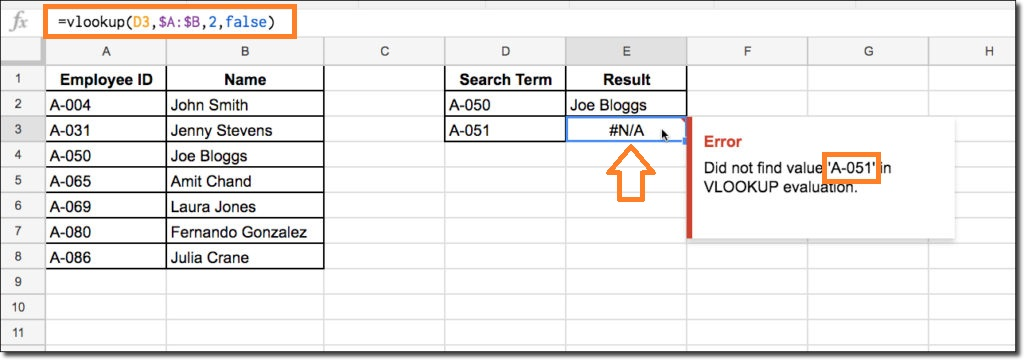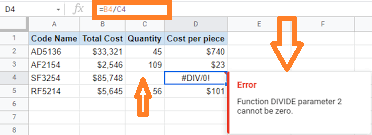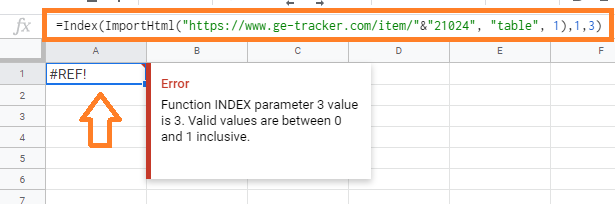## Formula Parse Error in Google Sheets and How to Fix Them With Examples

When working on Google Sheets, we use n number of formulas. You would certainly have faced an error in Google Sheets, even if you were an expert or a novice. The errors which are shown in Google Sheets don’t make us happy. Thus we need to fix the errors. However, in order to fix the errors on Google Spreadsheets, we must know what is causing the Parse error and how to fix it. In this article, let’s understand everything Formula Parse Error along with important Google Sheets tips. Read on to find out more.

## What is Formula Parse Error in Google Sheets?

When Google Sheets cannot understand your formula, a formulation parsing error occurs. There are several reasons which cause the Formula Parse error and a few of them are discussed below:

1. The formula could have a typo error.
2. The number expected for a specific function can be more or less than the number.
3. One or more entered parameters could be different than expected.
4. In your formula, cell references may be out of boundaries.
5. When trying to attempt mathematically impossible calculations.

There can be a number of other reasons, which make it hard for Google Sheets to meet the demands of your formulation. As such, an error message is returned.

### Types of Formula Parse Errors in Google Sheets

The types of formula parse errors in Google Sheets are explained below:

### N/A Error in Google Sheets

This error frequently happens when a formula requires a specific value that isn’t provided. The error message’s ‘N/A’ simply means ‘not available.’ To put it another way, the value is unavailable for the formula to use.

When you use lookup formulas like VLOOKUP in Google Sheets, you’ll frequently run into this issue.
If you’re looking for a value and it doesn’t exist in the range you’ve specified, you’ll most likely get a #N/A error.

The VLOOKUP function must find the value ‘A-051′ from the range A:B in the figure below. The function merely returns a #N/A function to signal that the value ‘has not been discovered’ because the value ‘A-051′ does not exist in the range.### How to Correct NA Error?

You can fix this by combining the formula with an IF statement that shows a custom message if the #N/A problem occurs. The IFERROR function is a very handy function for dealing with these kinds of problems.

### #DIV/0 Error in Google Sheets

When a number in the formula is divided by zero, an error happens. The function returns a #DIV/0 error since it’s an illogical mathematical operation. If you try to divide a value by a function or operation that returns a 0 value, you will get a #DIV/0 error.

In the following example, we are attempting to divide the number of the difference between the values in D4 and C4, which yields 0. Because dividing D4 by 0 is impossible, the calculation yields a #DIV/0 error.This Div error also occurs when you try to use the AVERAGE function on a range of blank cells, you’ll get this error. This occurs because the AVERAGE function must divide the SUM of the values by the number of values, which is equal to dividing by 0 if the range is blank.

### How to Correct Div Error in Google Sheets?

To correct this error, first determine why your denominator is evaluating a ‘0’ value. In the formula bar, select elements of the denominator to see what each part evaluates to. If it doesn’t work, see if any part of the denominator of the formula refers to a blank cell or range. If this is the case, you can either put in the required numbers in the cell or pick the formula’s required range.

### REF Error in Google Sheets

This error happens when your formula has an incorrect reference. There are various kinds of incorrect references such as:

• Missing Reference: when you use a cell that has since been deleted in a formula. When you delete a row or column in your worksheet, not just the value inside the cell, but the entire cell is deleted.
• Out of bounds lookup: If you use lookup formulae regularly, you’ve probably seen the #REF! error while trying to return a value outside of the ranges you’ve provided.
• Circular Dependency: When a circular dependency is found, such as when the formula references to itself, you’ll also get a #REF! error.### How to Fix #REF! Error in Google Sheets?

Find out what kind of #REF! mistake your formula has by reading the error message. If there are any missing references, the formula bar will show you the exact reference that is incorrect. The #REF! will be used to replace the problematic reference.

### Other Formula Parse Errors in Google Sheets

• #VALUE! Error: This is one of the most common errors that Google Sheets users encounter. It occurs when you perform mathematical operations on text strings in one or more cells.
• #NAME? Error: The #NAME? formula parse error indicates that your formula syntax is incorrect. A typo in one of your function names is the most likely cause of this problem.
• #NUM Error: In Google Sheets, this issue is uncommon. An invalid argument in a Google Sheets formula causes the #NUM! error.